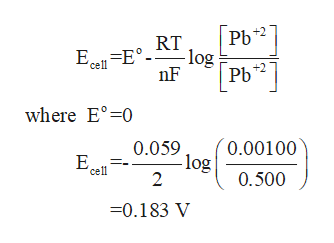# Calculate the cell potential (Ecell) for the following lead concentration cell at 298 K.[Pb2+] = 0.00100 M || [Pb2+] = 0.500 M

Question
123 views

Calculate the cell potential (Ecell) for the following lead concentration cell at 298 K.

[Pb2+] = 0.00100 M || [Pb2+] = 0.500 M

check_circle

Step 1

Given that,

Concentration of Pb+2 =0.00100M

Concentration of Pb+2 =0.500M

Step 2

The half cell reaction is

Step 3

Now, you calculate the...help_outlineImage TranscriptionclosePb РЬ RT EqE log nF cell +2 where E° 0 0.00100 log 2 0.059 Е. cell 0.500 -0.183 V fullscreen

### Want to see the full answer?

See Solution

#### Want to see this answer and more?

Solutions are written by subject experts who are available 24/7. Questions are typically answered within 1 hour.*

See Solution
*Response times may vary by subject and question.
Tagged in

### Chemistry